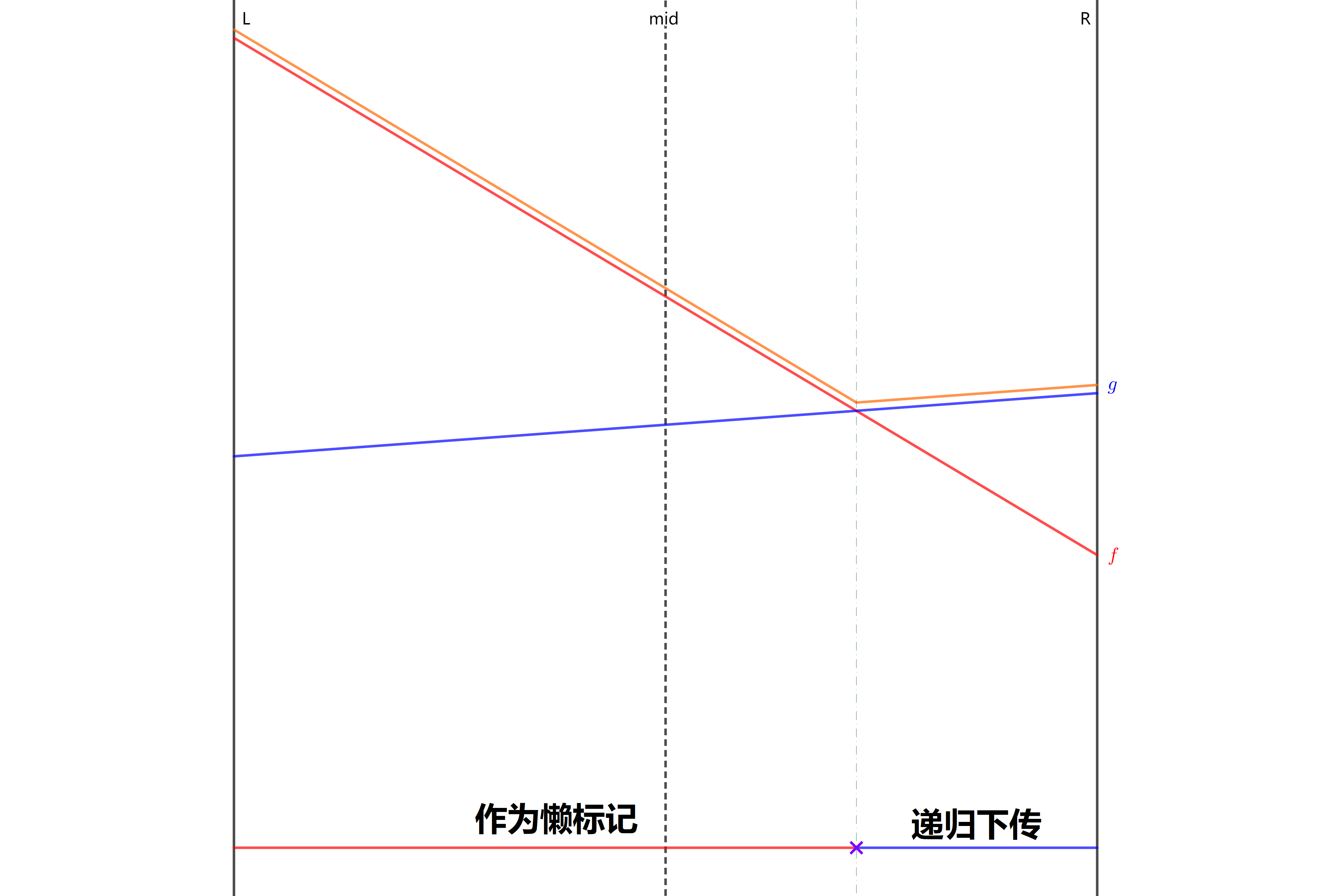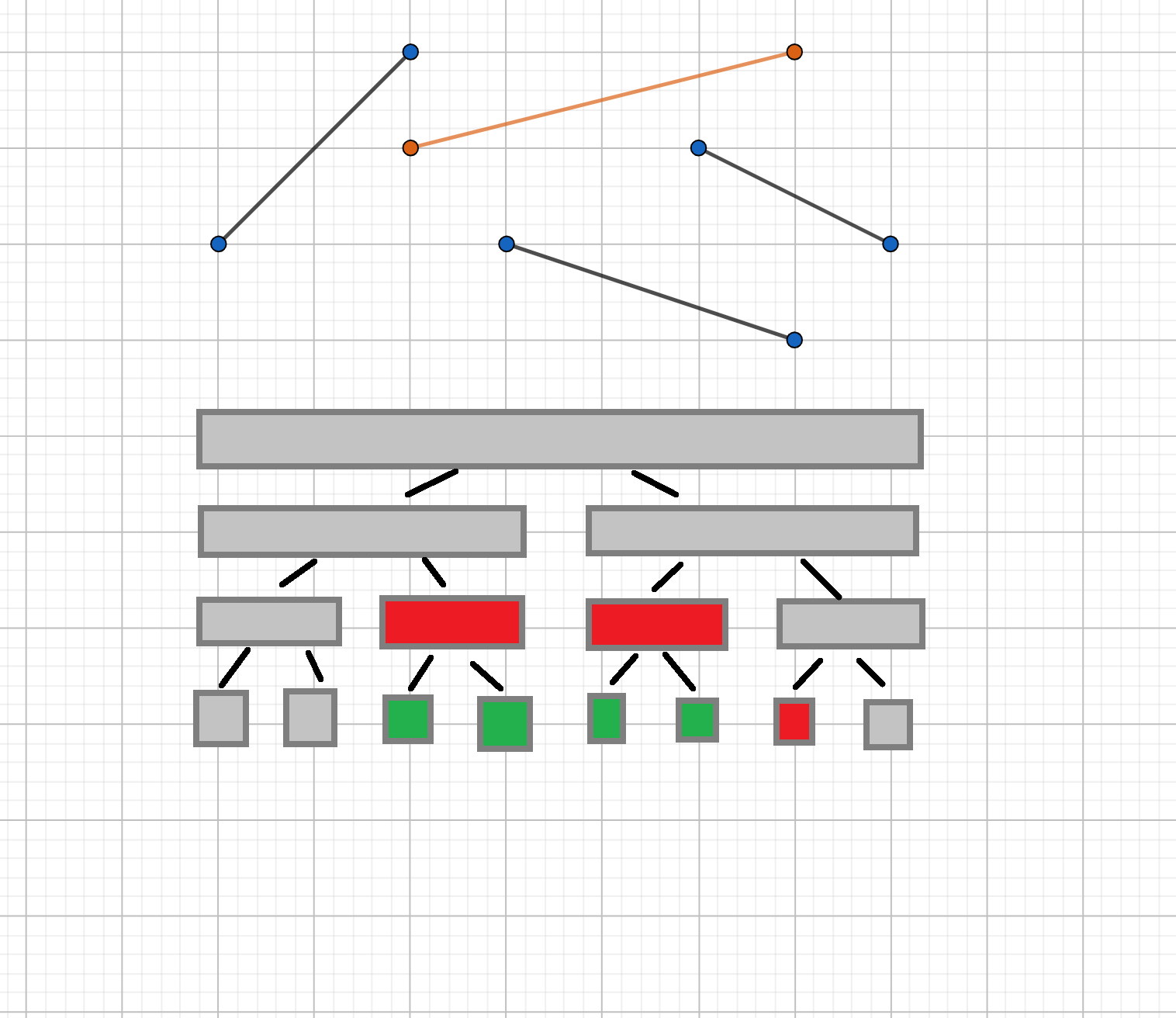# 李超线段树

## 引入

1. 在平面上加入一条线段。记第条被插入的线段的标号为，该线段的两个端点分别为2. 给定一个数，询问与直线相交的线段中，交点纵坐标最大的线段的编号（若有多条线段与查询直线的交点纵坐标都是最大的，则输出编号最小的线段）。特别地，若不存在线段与给定直线相交，输出## 过程

• 加入一个一次函数，定义域为• 给定，求定义域包含的所有一次函数中，在处取值最大的那个，如果有多个函数取值相同，选编号最小的。1. 若在左端点处更优，那么必然在左半区间中产生了交点，只有在左区间才可能优于，递归到左儿子中进行下传；
2. 若在右端点处更优，那么必然在右半区间中产生了交点，只有在右区间才可能优于，递归到右儿子中进行下传；
3. 若在左右端点处都更优，那么不可能成为答案，不需要继续下传。

  1 2 3 4 5 6 7 8 9 10 11 12 13 14 15 16 17 18 19 const double eps = 1e-9; int cmp(double x, double y) { // 因为用到了浮点数，所以会有精度误差 if (x - y > eps) return 1; if (y - x > eps) return -1; return 0; } //... void upd(int root, int cl, int cr, int u) { // 对线段完全覆盖到的区间进行修改 int &v = s[root], mid = (cl + cr) >> 1; if (cmp(calc(u, mid), calc(v, mid)) == 1) swap(u, v); int bl = cmp(calc(u, cl), calc(v, cl)), br = cmp(calc(u, cr), calc(v, cr)); if (bl == 1 || (!bl && u < v)) // 在此题中记得判线段编号 upd(root << 1, cl, mid, u); if (br == 1 || (!br && u < v)) upd(root << 1 | 1, mid + 1, cr, u); // 上面两个 if 的条件最多只有一个成立，这保证了李超树的时间复杂度 } 

  1 2 3 4 5 6 7 8 9 10 void update(int root, int cl, int cr, int l, int r, int u) { // 定位插入线段完全覆盖到的区间 if (l <= cl && cr <= r) { upd(root, cl, cr, u); // 完全覆盖当前区间，更新当前区间的标记 return; } int mid = (cl + cr) >> 1; if (l <= mid) update(root << 1, cl, mid, l, r, u); // 递归拆分区间 if (mid < r) update(root << 1 | 1, mid + 1, cr, l, r, u); }1 2 3 4 5 6 7 8 pdi query(int root, int l, int r, int d) { // 查询 if (r < d || d < l) return {0, 0}; int mid = (l + r) >> 1; double res = calc(s[root], d); if (l == r) return {res, s[root]}; return pmax({res, s[root]}, pmax(query(root << 1, l, mid, d), query(root << 1 | 1, mid + 1, r, d))); } 

[HEOI2013]Segment 参考代码
  1 2 3 4 5 6 7 8 9 10 11 12 13 14 15 16 17 18 19 20 21 22 23 24 25 26 27 28 29 30 31 32 33 34 35 36 37 38 39 40 41 42 43 44 45 46 47 48 49 50 51 52 53 54 55 56 57 58 59 60 61 62 63 64 65 66 67 68 69 70 71 72 73 74 75 76 77 78 79 80 81 82 83 84 85 86 87 88 89 90 91 92 93 94 95 96 #include #include #define MOD1 39989 #define MOD2 1000000000 #define MAXT 40000 using namespace std; typedef pair pdi; const double eps = 1e-9; int cmp(double x, double y) { if (x - y > eps) return 1; if (y - x > eps) return -1; return 0; } struct line { double k, b; } p; int s; int cnt; double calc(int id, int d) { return p[id].b + p[id].k * d; } void add(int x0, int y0, int x1, int y1) { cnt++; if (x0 == x1) // 特判直线斜率不存在的情况 p[cnt].k = 0, p[cnt].b = max(y0, y1); else p[cnt].k = 1.0 * (y1 - y0) / (x1 - x0), p[cnt].b = y0 - p[cnt].k * x0; } void upd(int root, int cl, int cr, int u) { // 对线段完全覆盖到的区间进行修改 int &v = s[root], mid = (cl + cr) >> 1; if (cmp(calc(u, mid), calc(v, mid)) == 1) swap(u, v); int bl = cmp(calc(u, cl), calc(v, cl)), br = cmp(calc(u, cr), calc(v, cr)); if (bl == 1 || (!bl && u < v)) upd(root << 1, cl, mid, u); if (br == 1 || (!br && u < v)) upd(root << 1 | 1, mid + 1, cr, u); } void update(int root, int cl, int cr, int l, int r, int u) { // 定位插入线段完全覆盖到的区间 if (l <= cl && cr <= r) { upd(root, cl, cr, u); return; } int mid = (cl + cr) >> 1; if (l <= mid) update(root << 1, cl, mid, l, r, u); if (mid < r) update(root << 1 | 1, mid + 1, cr, l, r, u); } pdi pmax(pdi x, pdi y) { // pair max函数 if (cmp(x.first, y.first) == -1) return y; else if (cmp(x.first, y.first) == 1) return x; else return x.second < y.second ? x : y; } pdi query(int root, int l, int r, int d) { // 查询 if (r < d || d < l) return {0, 0}; int mid = (l + r) >> 1; double res = calc(s[root], d); if (l == r) return {res, s[root]}; return pmax({res, s[root]}, pmax(query(root << 1, l, mid, d), query(root << 1 | 1, mid + 1, r, d))); } int main() { ios::sync_with_stdio(false); int n, lastans = 0; cin >> n; while (n--) { int op; cin >> op; if (op == 1) { int x0, y0, x1, y1; cin >> x0 >> y0 >> x1 >> y1; x0 = (x0 + lastans - 1 + MOD1) % MOD1 + 1, x1 = (x1 + lastans - 1 + MOD1) % MOD1 + 1; y0 = (y0 + lastans - 1 + MOD2) % MOD2 + 1, y1 = (y1 + lastans - 1 + MOD2) % MOD2 + 1; if (x0 > x1) swap(x0, x1), swap(y0, y1); add(x0, y0, x1, y1); update(1, 1, MOD1, x0, x1, cnt); } else { int x; cin >> x; x = (x + lastans - 1 + MOD1) % MOD1 + 1; cout << (lastans = query(1, 1, MOD1, x).second) << endl; } } return 0; }